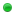4 word combinations from a short list of wordsDipperdoorsJunior MemberPosts: 2 Threads: 1 Joined: Dec 2017 12-23-2017, 01:49 PM Using a list of 1500 common nouns, I need to try all combinations of exactly four nouns and their plurals (add s) to crack an MD5.  wordlist: fruit apple pear banana turkey tree photo plant example passwords fruitsapplepearbanana turkeytreesphotoplant no capitals, so spaces. I've looked around, but can only figure out how to do combine two. Any pointers?philsmdI'm philPosts: 1,372 Threads: 12 Joined: Feb 2013 12-23-2017, 02:22 PM you could use someting like this (a perl script) and change the words if you need: Code:```#!/usr/bin/env perl use strict; use warnings; my @words = (   'fruit',   'apple',   'pear',   'banana',   'turkey',   'tree',   'photo',   'plant' ); my \$count = scalar (@words); for (my \$i = 0; \$i < \$count; \$i++) {   for (my \$j = 0; \$j < \$count; \$j++)   {     next if (\$j == \$i);     for (my \$k = 0; \$k < \$count; \$k++)     {       next if (\$k == \$i);       next if (\$k == \$j);       for (my \$l = 0; \$l < \$count; \$l++)       {         next if (\$l == \$i);         next if (\$l == \$j);         next if (\$l == \$k);         # 0 plural         print \$words[\$i]       . \$words[\$j]       . \$words[\$k]       . \$words[\$l]       . "\n";         # 1 plural         print \$words[\$i] . "s" . \$words[\$j]       . \$words[\$k]       . \$words[\$l]       . "\n";         print \$words[\$i]       . \$words[\$j] . "s" . \$words[\$k]       . \$words[\$l]       . "\n";         print \$words[\$i]       . \$words[\$j]       . \$words[\$k] . "s" . \$words[\$l]       . "\n";         print \$words[\$i]       . \$words[\$j]       . \$words[\$k]       . \$words[\$l] . "s" . "\n";         # 2 plurals (1st word)         print \$words[\$i] . "s" . \$words[\$j] . "s" . \$words[\$k]       . \$words[\$l]       . "\n";         print \$words[\$i] . "s" . \$words[\$j]       . \$words[\$k] . "s" . \$words[\$l]       . "\n";         print \$words[\$i] . "s" . \$words[\$j]       . \$words[\$k]       . \$words[\$l] . "s" . "\n";         # 2 plurals (2nd word)         print \$words[\$i]       . \$words[\$j] . "s" . \$words[\$k] . "s" . \$words[\$l]       . "\n";         print \$words[\$i]       . \$words[\$j] . "s" . \$words[\$k]       . \$words[\$l] . "s" . "\n";         # 2 plurals (3rd word)         print \$words[\$i]       . \$words[\$j]       . \$words[\$k] . "s" . \$words[\$l] . "s" . "\n";         # 3 plurals (1st word)         print \$words[\$i] . "s" . \$words[\$j] . "s" . \$words[\$k] . "s" . \$words[\$l]       . "\n";         print \$words[\$i] . "s" . \$words[\$j] . "s" . \$words[\$k]       . \$words[\$l] . "s" . "\n";         print \$words[\$i] . "s" . \$words[\$j]       . \$words[\$k] . "s" . \$words[\$l] . "s" . "\n";         # 3 plurals (2nd word)         print \$words[\$i]       . \$words[\$j] . "s" . \$words[\$k] . "s" . \$words[\$l] . "s" . "\n";         # 4 plurals         print \$words[\$i] . "s" . \$words[\$j] . "s" . \$words[\$k] . "s" . \$words[\$l] . "s" . "\n";       }     }   } }``` The only "probem" is that it will increase very fast the more words there are in the array/dict.undeathSneaky BastardPosts: 1,760 Threads: 11 Joined: Jul 2010 12-23-2017, 02:32 PM You can create a dict of two combined nouns using either combinator or hashcat's stdout and then run a combinator attack with that new dict.devilsadvocateMemberPosts: 93 Threads: 31 Joined: Aug 2014 12-23-2017, 05:29 PM You can create a short wordlist and then feed it to prince processor with "--elem-cnt-min=4 --elem-cnt-max=4" Interesting perl solution though.DipperdoorsJunior MemberPosts: 2 Threads: 1 Joined: Dec 2017 12-24-2017, 08:30 AM (12-23-2017, 02:22 PM)philsmd Wrote: The only "probem" is that it will increase very fast the more words there are in the array/dict. Perl script is interesting, thx but the size a big problem with the dictionary (list is n=1500, so I think the total number of entries with plurals is 3000^4 or something like that). Thx though, I had fun with it, haven't looked at perl before. « Next Oldest | Next Newest »